# how to create own surface profile service?

1646
19
04-30-2010 01:53 AM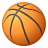New Contributor II
Hi

The GPservice used to get surface profile in ESRI samples is " http://sampleserver2.arcgisonline.com/ArcGIS/rest/services/Elevation/ESRI_Elevation_World/GPServer/P..."

How do i create my own service to get the same result with my data than ESRI sample data..

Or is it possible to get surface profile from my data using esri's profileservice? cos when i tried i ended up getting errors. im confused whether its my data or service.. im using DEM.

could somebody please give solution for this please. Im totaly messed up.
Tags (3)
19 Replies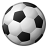New Contributor
I would like to create my own service as well.  Any word on this?

AndrewNew Contributor II
why is it that whenever a question is posted on surface elevation profile service creation for oneself,  there is no answer !!! i mean is it something which is not possible ?? kindly acknowledge..Occasional Contributor
I am also very interested to create my own surface profile GPService.

Did anyone get some more information here?Occasional Contributor
i just have to chime in as well. this would be nice to know.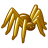Occasional Contributor III
I would like to know how to use ESRI's profile service using javascript.  I am actually trying to create a slope calculator.  However to try to answer other questions.  I would think you could create a geometry service (which usually accounts for x,y coordinates and then be able to calculate a z value at that point based on your data)  In a profile your line would have several x,y coordinates and calculate the z values at each one of those x,y points.  The result would be your profile.....

Now anyone know how to write javascript to use ESRI's profile service using Javascript API?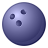by
New Contributor
TTT!

I would also like to see an answer to this. Is ESRI actively ignoring these?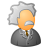New Contributor
I also would like to know how it's done?
Seems no one from ESRI interested in this subject.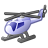New Contributor III
ditto,

come on esri .. chime in for f(**( sake ... even if the answer is no.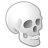New Contributor III
I managed to hack this together at one point, but found it was too slow for a web service.  I rewrote the script using gdal for raster access and it was hundreds of times faster.  The code for the arcpy script is below, with an example output graph.  The commented code was for grabbing points from a feature set (for a toolbox or gp service).  This ugly, but it works.  disclaimer: This is hacked, old and ugly.  sorry.

```from polyline import *
import matplotlib.pyplot as plt

def fetch_cell_value(x, y):
xy_string = "{0} {1}".format(x, y)
band = "1"

arcpy.GetCellValue_management(r, xy_string, band)
results = arcpy.GetMessages()
lines = results.split('\n')
value = float(lines)
return value

#for arc script
#fs = arcpy.GetParameter(0)
#rows = arcpy.SearchCursor(fs)

# Assume feature set has only one feature and one shape.
#row = rows.next()
#shape = row.getValue("SHAPE")
#arcpoints = shape.getPart(0)

#points = list()
#for p in arcpoints:
#    points.append([p.X, p.Y])

raster = "c:/data/someraster.tif"
r = arcpy.Raster(raster)

#test points
points = [[290626.474, 4875792.8594],
[282358.29,4863026.666],
[297836.3843, 4870699.62]]

p = poly_line(points)

n_points = 100
#if p.length() / n_points < cell_size:
#    skip = cell_size
#else:
#    skip = int(p.length() / n_points)
skip = p.length() / n_points

dists = list()
i = 0
while i * skip < p.length():
dists.append(i * skip)
i += 1

values = list()
for d in dists:
x,y = p.d_line(d)
v = fetch_cell_value(x,y)
values.append(v)
print("Time:{0}".format(time.clock() - start))

plt.plot(dists, values)
plt.xlabel("Distance (m)")
plt.ylabel("Elevation (m)")
plt.title("Elevation Profile")
plt.grid(True)
plt.show()
```

The poly_line is a little class I wrote to handle densifying lines.  It assumes euclidean geometry (planar) so doesn't handle earth curvature.  You could probably use some type of densify function for an arc type polyline and use the points in the shape.  I didn't try it.

poly_line:

```import math

##
# Class representing a segment of a polyline
#
class segment:
p1 = [0,0]
p2 = [0,0]
##
# @brief Construct a segment
#
def __init__(self, p1, p2):
self.p1 = p1
self.p2 = p2
##
# @brief Get the length of the segment
#
# @todo Test for /0
#
def length(self):
a = self.p2 - self.p1
b = self.p2 - self.p1
l = math.hypot(a,b)
return l
##
# @brief Get the slope of the segment
#
# @todo Test for /0
#
def m(self):
a = self.p2 - self.p1
b = self.p2 - self.p1
m = a / b
return m
##
# @brief Get the y intercept of the segment
#
# @return y intercept
def b(self):
b = self.p2 - (self.m() * self.p2)
return b
##
# @brief Get the coordinate of a point some distance from point 1
#
# @param d distance from point 1
# @return x and y coordinate
def d_line(self, d):
if d == 0:
return self.p1, self.p1
if d > self.length():
return None
m = self.m()
u = d / math.sqrt(m * m + 1)
if self.p2 < self.p1:
u = -u
v = (m * d) / math.sqrt(m * m + 1)
if self.p2 < self.p1:
v = -v
return (self.p1 + u, self.p1 + v)
##
# Class representing a polyline composed of segments
#
# @see segment
#
class poly_line:
segments = list()
def __init__(self, points):
for i in range(len(points) - 1):
s = segment(points, points[i+1])
self.segments.append(s)
##
# @brief Get the length of all the segments
#
#
def length(self):
l = 0.0
for s in self.segments:
l += s.length()
return l
##
# @brief Get the coordinate of a point some distance from the polyline
# origin
#
# @param d distance from the origin
# @return x and y coordinate for the point
#
def d_line(self, d):
if d == 0:
return self.segments.d_line(0)
l = 0.0
for s in self.segments:
if l >= d:
break
dd = d - l
l += s.length()
seg = s
return seg.d_line(dd)
```

I just ran it and wrote the attached output, but no guarentees it will work for anyone.  I think that the service ESRI wrote used lower level raster access, because of it's speed.  I got comparable speed with the gdal version.

Sorry if I didn't help, but it is possible.  I stopped working on it, but it may help others.  I will watch the thread and try to answer questions.

kss